# Control System – Frequency Response Analysis

We have learned the time response analysis in detail (in my series of previous posts) , in which we subjected a system to various inputs i.e step, ramp, parabolic etc.

All those inputs were functions of time ( and not frequency).By frequency response , we mean the steady-state response of a system when subjected to a sinusoidal input.In this method, we vary the input frequency over a certain range and study the resulting response.

Consider a linear system , we subject a sinusoidal input to this system (which is linear),

r(t)  =  A sin (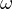t)  ,

Under steady state, the output of the system c(t) will be of the form : c(t) =  B sin (t + φ )  ,

In other words, when a sinusoidal input is fed to a linear system , the output of the system is also a sinusoidal signal of the same frequency but different in magnitude and phase.The magnitude and phase relationship between the sinusoidal input and the steady state output is known as the frequency response.

Methods used in Frequency response :

We have two plots : Magnitude and phase plots. In both these the variable is the angular frequency ‘‘ . The following techniques are used widely:

• Bode Plots: There are two separate plots : (i) Magnitude (ii) Phase , both versus frequencyin logarithmic value.
• Polar and Nyquists plots : It is a single plot of magnitude versus phase angle as ω is varied from zero to infinity in polar co-ordinates.

Disadvantages of Frequency Response methods :

• The methods which are considered for obtaining frequency response are outdated as compared to extensive methods developed for modelling and simulations.
• These techniques are basically applied to linear systems.

Derivation  of Resonant frequency and Resonant Peak frequency

The two  important specifications of frequency response are Resonant peak (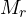) and Resonant Frequency (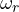) . We shall now derive them.

Consider a standard second order system .

Here,  G(s) =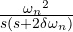,    H(s) = 1

The closed loop transfer function is

T(s) =   C(s)/R(s)     =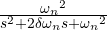We convert the equation to the frequency response by replacing s by j,

Hence, T(j)   =  C(j)/R(j)         =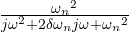,

Dividing  numerator and denominator by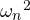, and combining the real parts,

C(j)/R(j)  =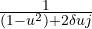( by letting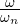=  u  ) ,

This term is complex and hence has magnitude and phase ,

Magnitude (M) =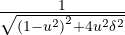,

We know that resonant frequency  at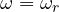,  magnitude is maximum . Using maxima theorem of derivatives, we get the  maximum magnitude when,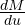= 0 ,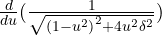=  0 ,=    [ (-1/2)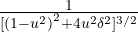] [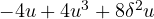]   =  0

Of the two terms , the first term cannot be zero.

Hence,=  0 ,

On solving, we get   u = 0  or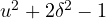= 0

Now,=  u   cannot be zero,

So,= 0 ,

Hence , u  =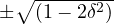,

Again=  u   > 0 ,

So, u  =,    Also=  u  =,

Then=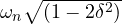( here  we have,)

Hence ,==  Resonating Frequency

Now, Resonant peak can be easily found out by  substituting  the value of u in the equation of M,

We know  M   =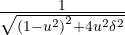=on putting the value of ‘u’   ,

Thus , on solving, we get=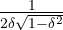,

From the equations of both i.eand, it can be concluded that:

• As ‘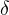‘  approaches to zero ,approaches to ∞ (infinity) andapproaches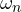.
• For 0 << 0.707 ,is greater than 1, and<.
• For> 0.707 , there is no resonant peak.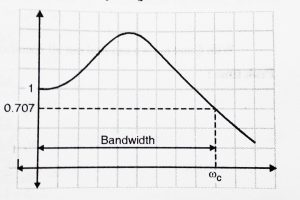Bandwidth:

Apart from resonance frequency and  Resonant peak , there is one more specification in the frequency response which is extremely important – bandwidth.

It is the range of frequencies  upto the cutoff frequency (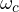)  beyond which  the magnitude  ( M ) drops down by 3 db ( 0.707)  below zero frequency level.  Let us derive formula for Bandwidth.From above derivation we can directly write the value of magnitude ‘M’ :

Magnitude (M) =  |T(j)|  =,

Now for |T(j)|, a drop by 3 dB is calculated as shown below:

20 log|T(j)|  = -3   ,

20log [] = -3 ,

-20 log (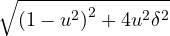)  =  -3

On solving, by taking antilog both sides,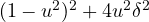=  1.995 ( can be round off to 2 )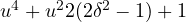=  0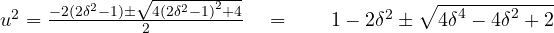We take square root both the sides,

u =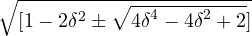(since u > 0 , we take only positive value)

Now u =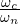=,=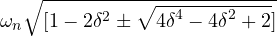,

Hereis the cut off frequency . Hence the Bandwidth is 0 –.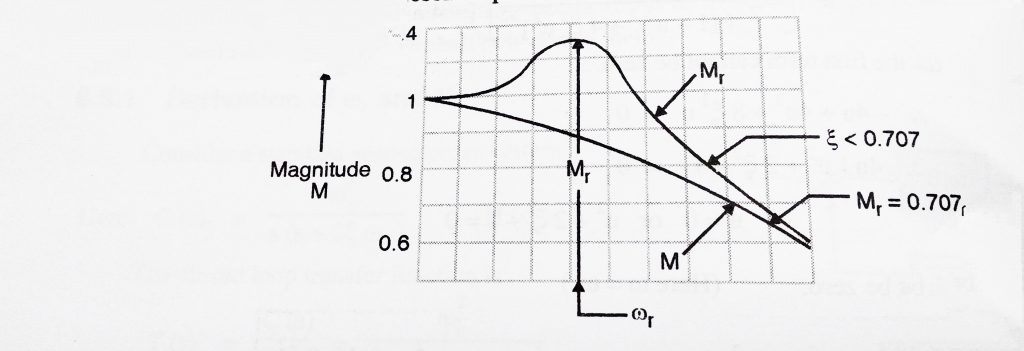Thus Frequency Response of a controller method clarifies the nature of the process when signals are put into it.  So we generally do it by analyzing the ‘magnitude’ and ‘phase’separately. The magnitude response of a machine will provide us a concept of this gain/attenuation the system exerts to a specified frequency.

The stage response informs us by how much a Specific frequency Part of the signal May Be delayed/advanced from the system. In my next post, we will see various methods to analyze the frequency response of any system in detail. First we will learn ‘Bode Plot’ technique followed by polar and Nyquist plot.So stay tuned.